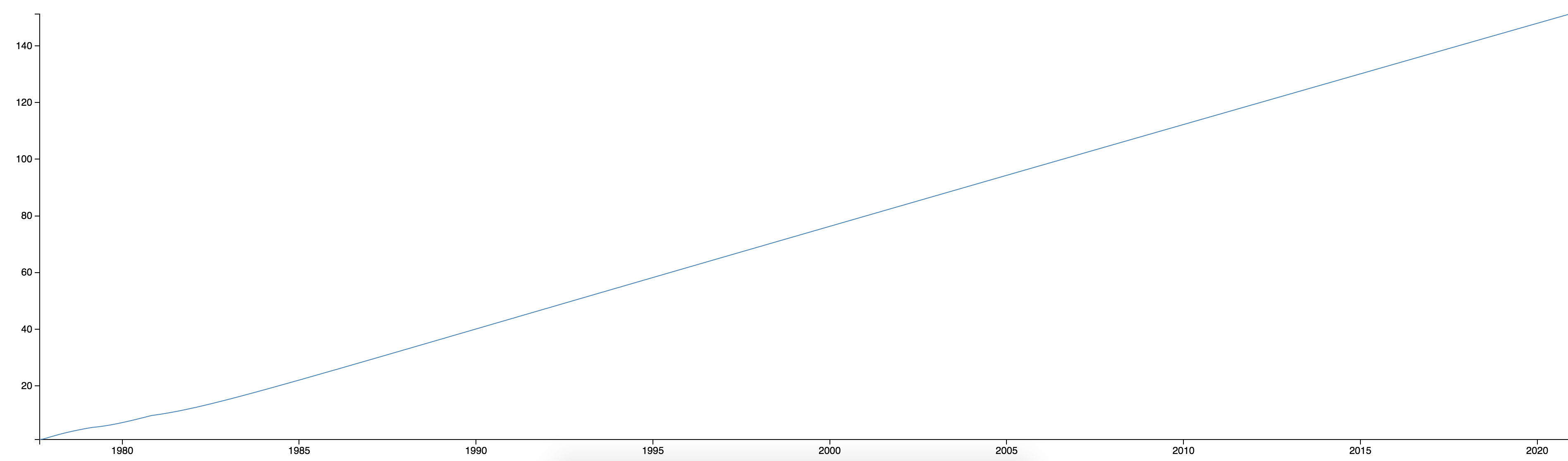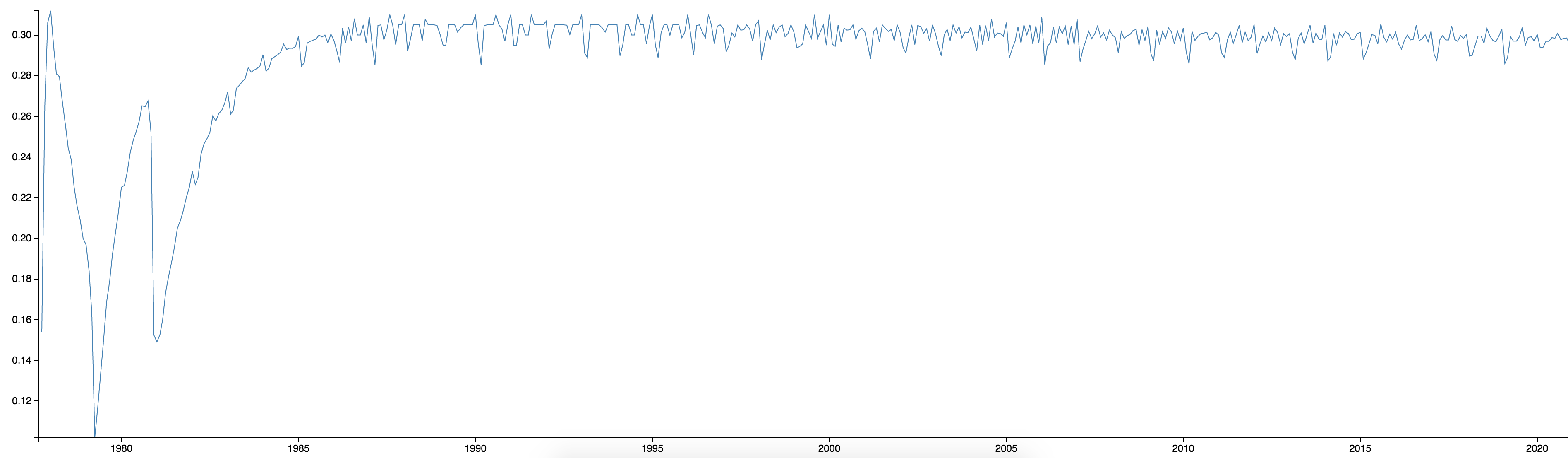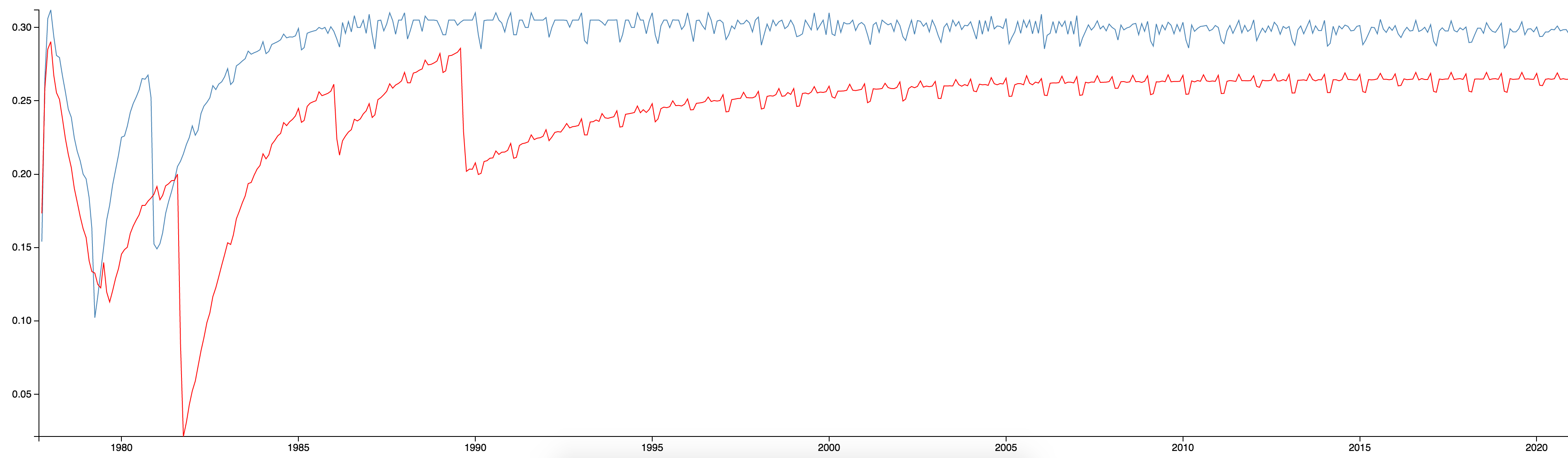# Out of this world aggregations

One of the most visible features coming in 2.0 are the Pipeline Aggregations. This is an extension of the current Aggregations framework, to allow additional computations to be performed on top of the results of aggregations. This allows users to ask questions such as “What is the maximum average monthly price?” from a date histogram and “How many new users are signing up each day?” from a date histogram showing total user count each day.

Internally these aggregation are run after the other aggregations have completed on the coordinating node. This means Pipeline Aggregators are able to use the final results of their sibling aggregations but are not able to go back to the shards to query the index. The first of these new types are:

In this post I will show some of these new aggregations in action. To help demonstrate, I have downloaded spacecraft trajectory data from NASA’s Helioweb site for the Voyager 1 and Voyager 2 spacecraft. Each document in my index represents the Solar Ecliptic position of one of the spacecraft on a particular day. The data spans from the launch of the Voyager missions in September 1977 to the present day and also includes projected trajectories up to 2020. Below is an example of a document in Elasticsearch:

{
"_index": "helioweb",
"_type": "voyager1",
"_id": "1977-257",
"_score": 1,
"_source": {
"seLon": 353.8,
"objectName": "voyager1",
"seLat": 0.2,
"year": 1977,
"date": "1977-09-14T10:28:25.178Z",
"dayOfYear": 257
}
}


seLat and seLon are the latitude and longitude of the object in the Solar Ecliptic Coordinate System respectively, and radAU is the radial distance of the object from the Sun measured in Astronomical Units. In this post we will mainly be concentrating on the date, objectName, and radAU fields.

So first let’s plot the average radial distance of Voyager 1 over time. This can be done with the existing aggregations by defining the following aggregations:

{
...
"aggs": {
"histo": {
"date_histogram": {
"field": "date",
"interval": "month"
},
"aggs": {
"avg_distance": {
"avg": {
}
}
}
}
}
}


The graph we get from the results looks pretty uninteresting:Basically all we can find out from this is that since launch Voyager 1 has been travelling further and further away from the Sun. Not exactly surprising for a long distance space probe!

But what does Voyager 1’s radial speed over time look like? This isn’t something you can currently do in Elasticsearch. The indexed documents only contain the radial distance of Voyager 1 so its speed cannot be plotted directly. With the new Derivative Aggregation we can take the derivative of that distance to calculate the radial speed each month:

{
...
"aggs": {
"histo": {
"date_histogram": {
"field": "date",
"interval": "month"
},
"aggs": {
"avg_distance": {
"avg": {
}
},
"speed": {
"derivative": {
"buckets_path": "avg_distance"
}
}
}
}
}
}


Pipeline aggregations look very similar to the other aggregations in the request, but instead of having a field parameter to reference which field in the document they will work on, they have a bucket_path parameter which references which aggregation to use in their calculation. The resulting aggregation tree for the above request looks like this:

{
...
"aggregations": {
"histo": {
"buckets": [
{
"key_as_string": "1977-09-01T00:00:00.000Z",
"key": 241920000000,
"doc_count": 25,
"avg_distance": {
"value": 1.0328
}
},
{
"key_as_string": "1977-10-01T00:00:00.000Z",
"key": 244512000000,
"doc_count": 31,
"avg_distance": {
"value": 1.1867741935483873
},
"speed": {
"value": 0.15397419354838737
}
},
…
]
}
}
}


You can see that the Derivative Aggregation has added a new value speed to the all but the first bucket. The first bucket is left empty as we require two values to compute the derivative, the current value and the previous value. Since there is no previous value for the first bucket, we can’t compute a derivative for it.

Plotting the speed against time gives us a much more interesting graph:Here we can see that Voyager 1’s radial speed dropped significantly twice, once around 1979 and once around 1981. Looking at the timeline for the Voyager mission we can see that these points coincide with the closest approach of Voyager 1 with Jupiter and Saturn.

We can actually use the Min Bucket Aggregation to determine which month Voyager 1 was travelling at its slowest radial speed using the following:

{
...
"aggs": {
"histo": {
"date_histogram": {
"field": "date",
"interval": "month"
},
"aggs": {
"avg_distance": {
"avg": {
}
},
"speed": {
"derivative": {
"buckets_path": "avg_distance"
}
}
}
},
"min-speed": {
"min_bucket": {
"buckets_path": "histo > speed"
}
}
}
}


Which returns a new aggregation alongside the histogram showing the minimum value for the slowest radial speed and the bucket keys of the bucket which have this minimum value:

{
...
"aggregations": {
"histo": {
"buckets": [
...
]
},
"min-speed": {
"value": 0.10212903225806436,
"keys": [
"1979-04-01T00:00:00.000Z"
]
}
}
}


So the slowest radial speed was in April 1979 which matches the Voyager timeline as the closest approach to Jupiter was during March 1979. Previously this would have required the client application to parse all the buckets, keeping track of the minimum value.

We can show the radial speed of both Voyager 1 and Voyager 2 in the same response by using the filter aggregation to show the average radial distance of Voyager 1 and Voyager 2 and then use two derivative aggregations, one referencing the Voyager 1 distance and the other referencing the Voyager 2 distance:

{
"aggs": {
"histo": {
"date_histogram": {
"field": "date",
"interval": "month"
},
"aggs": {
"voyager_1": {
"filter": {
"term": {
"objectName": "voyager1"
}
},
"aggs": {
"avg_distance": {
"avg": {
}
}
}
},
"voyager_2": {
"filter": {
"term": {
"objectName": "voyager2"
}
},
"aggs": {
"avg_distance": {
"avg": {
}
}
}
},
"voyager_1_speed": {
"derivative": {
"buckets_path": "voyager_1>avg_distance"
}
},
"voyager_2_speed": {
"derivative": {
"buckets_path": "voyager_2>avg_distance"
}
}
}
}
}
}


Now, the buckets_path references the avg_distance metric inside each of the filter aggregations using the buckets_path syntax. We can now plot the radial speed of Voyager 1 (blue line) and Voyager 2 (red line):Again from looking at the timeline for the Voyager mission we can see that the drops in radial speed of Voyager 2 coincide with its closest encounters with Jupiter, Saturn, Uranus and Neptune.

Since pipeline aggregations happen right at the end of the aggregation phase, they can be chained together (hence the name pipeline). So to calculate the radial acceleration of the Voyager 1 spacecraft we can just calculate the derivative of the speed (which we calculated in the previous pipeline aggregation) by adding the following aggregation to the request:

{
...
"voyager_1_acceleration": {
"derivative": {
"buckets_path": "voyager_1_speed"
}
}
…
}


Here, instead of referencing a metric aggregation we are referencing the derivative aggregation voyager_1_speed. This will add an aggregation called voyager_1_acceleration to the response for all but the first 2 buckets (since we need two values for voyager_1_speed to calculate the acceleration).

These are just the first pipeline aggregations. We are currently working to add more pipeline aggregations to this list and there is a growing list of other ideas for possible pipeline aggregators including one that can be used to filter out buckets which do not meet a specified criteria (using this aggregation you could, for example, ask for only histogram buckets where the average speed is greater than a threshold value). We would also appreciate any feedback you have on the current pipeline aggregations and your suggestions for other pipeline aggregations that we could add in the future.

Happy exploring!